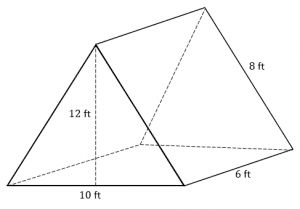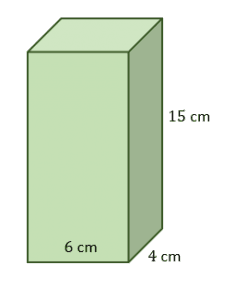# Surface Area of a Prism – Explanation & Examples

The total surface area of a prism is the sum of areas of its lateral faces and its two bases.

In this article, you will learn how to find the total surface area of a prism by using the surface area of a prism formula.

To recall, a prism is a 3-dimensional polyhedron with two parallel and congruent bases, which are connected by lateral faces. A prism is named according to the shape of the polygonal bases. In a prism, the lateral faces, which are parallelograms, are perpendicular to the polygonal bases.

## How to Find the Surface Area of a Prism?

• To find the total surface area of a prism, you need to calculate the area of two polygonal bases, i.e., the top face and bottom face.
• And then calculate the area of lateral faces connecting the bases.
• Add up the area of the two bases and the area of the lateral faces to get the total surface area of a prism.

### Total surface area of a prism formula

Since we know the total surface area of a prism is equal to the sum of all its faces, i.e., the floor, walls, and roof of a prism. Therefore, the surface area of a prism formula is given as:

Total surface area of a prism = 2 x area of the base + perimeter of the base x Height

TSA = 2B + ph

Where TSA = Total surface area of a prism

B = Base area

p = perimeter of the base

h = height of the prism

Note: The formula to find the base area (B) of a prism depends on the base’s shape.

Let’s solve a few example problems involving the surface area of different types of prisms.

Example 1

The dimensions of a triangular prism are given as follows:

Apothem length of the prism, a = 6 cm

Base length = 4 cm

height of the prism, h = 12 cm

The other two sides of the triangular base are 7 cm each.

Find the total surface area of the triangular prism.

Solution

By the formula,

TSA = 2 x area of the base + perimeter of the base x Height

Since the base is a triangle, then the base area, B =1/2 ba

=1/2 x 4 x 6

= 12 cm2.

Perimeter of the base, p = 4 + 7 + 7

= 18 cm

Now substitute the base area, height, and perimeter in the formula.

TSA = 2B + ph

= 2 x 12 + 18 x 12

= 24 + 216

= 240 cm2

Therefore, the total surface area of the triangular prism is 240 cm2.

Example 2

Find the total surface area of a prism whose base is an equilateral triangle of side 8 cm and height of the prism is 12 cm.

Solution

Given:

Height of the prism, h = 12 cm

The base is an equilateral triangle of side 8 cm.

By Pythagorean theorem, the apothem length, a of the prism is calculated as:

a = √ (82 – 42)

= √ (64 – 16)

= √ 48 = 6.93

Thus, the apothem length of the prism is 6.93 cm

Base area, B = ½ b a

= ½ x 8 x 6.93

= 27.72 cm2

Perimeter of the base = 8 + 8 + 8

= 24 cm

TSA = 2B + ph

= 2 x 27.72 + 24 x 12

= 55.44 + 288

= 343.44 cm2.

Hence, the total surface area of the prism is 343.44 cm2.

Example 3

The apothem length, base length, and height of a pentagonal prism are 10 cm. 13 cm, and 19 cm, respectively. Find the total surface area of the pentagonal prism.

Solution

The formula for the total surface area of a pentagonal prism is given by;

TSA = 5ab + 5bh

Where

By substitution, we have,

TSA = 5 x 10 x 13 + 5 x 13 x 19

= 650 +1235

= 1885 cm2

Thus, the total surface area of the pentagonal prism is 1885 cm2

Example 4

A rectangular prism of dimensions, length = 7 in, width = 5 in and height = 3 in is to be painted. If the painting cost is $50 per square inch, find the total cost of painting all faces of the prism. Solution First, calculate the total surface area of the prism Surface area of a rectangular prism = 2h (l +b) = 2 x 3 (7 + 5) = 6 x 12 TSA = 72 in2 The total cost of painting the prism = TSA x cost of painting = 72 x 50 =$3,600

Thus, the cost of painting the rectangular prism is $3,600 Example 5 Find the total surface area of a hexagonal prism whose apothem length, base length, and height are given as 7 m, 11 m, and 16 m, respectively. Solution The total surface area formula for a hexagonal prism is given as: TSA = 6ab + 6bh Substitute. TSA = 6 x 7 x 11 + 6 x 11 x 16 = 462 + 1056 =1518 m2 Example 6 Calculate the total surface area of an isosceles trapezoid whose parallel sides of the base are 50 mm and 120 mm and legs of the base are 45 mm each, the height of the base is 40 mm, and the height of the prism is 150 mm. Solution The total surface area of a trapezoid prism = 2B + ph Base area (B) of a trapezoid = 1/2h (b1 + b2) = ½ x 40(50 + 120) = 20 x 170 = 3400 mm2 Perimeter (p) of the base = 50 + 120 + 45 + 45 = 260 mm Now, substitute into the formula. TSA = 2 x 3400 + 260 x 150 = 6,800 + 39,000 = 45,800 mm2 ### Practice Questions 1. What is the total surface area of the triangular prism shown below?2. What is the total surface area of the rectangular prism shown below?3. A tank needs to be painted and it has a shape of a rectangular prism with the following dimensions: • Length =$12$in • Width =$8$in • Height =$5$in If the painting cost is$\$36$ per square inch, what is the total cost of painting all faces of the prism?

4. What is the total surface area of a square pyramid with the following dimensions?
• A square base with a length of $24$ ft.
• A height of $16$ ft.

5. If a cube has a total surface area of $150$ squared feet, what is its side length?